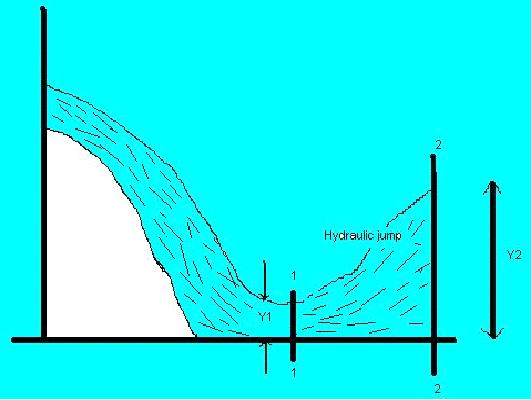Feb 26, 2016

Feb 26, 2016

Feb 26, 2016

# Calculation, Effects & Applications of Hydraulic Jump

## Definition

Hydraulic jump is the phenomenon of open channels. Before defining the hydraulic jump it is necessary to describe the terms "super critical flow" and "sub critical flow" in order to clearly understand that "what is hydraulic jump" Hydraulic jump usually occurs in super critical flow.

In order to describe the super critical flow and sub critical flow, there is need to define the Froude number. Froude number can be defined as the velocity divided by the square root of gravitational constant multiplied by the depth. It is dimensionless quantity.

`F = V/ vG x D`

Where V is velocity of water, D is depth of flow of water and G is gravitational constant. Froude number is an indicator of resistance of object moving in the water. If the Froude number is less than 1, then the flow of water is said to be as the sub critical flow. While if the Froude number is greater than 1, then the flow of water is said to be as the super critical flow. If it is equal to 1, then flow is said to be as the critical flow. As sub critical flow of water is reflection of slow moving deep water. On the other hand super critical flow is indicator of fast moving shallow water. Super critical flow is unstable form of flow whiles the sub critical is stable one

Now move toward the hydraulic jump, it can be defined as rise in the level of water when the water transforms its super critical form into the sub critical one i.e. from unstable state to the stable one. This phenomenon can be observed on spillways of the dam. As it's the nature of water to gain its stability after it is being unstable. The place where hydraulic jump occurs, a lot of energy is dissipated in the form of heat energy. We can say that hydraulic jump is dissipator of extra energy of water. This phenomenon is usually used in spillways of the dam to reduce the erosive power of super critical flow. Otherwise it will affect the dam structure.

## Calculation of hydraulic jump:

Height of hydraulic jump can be calculated by the formula:

`Y2 - Y1`Where Y1 is depth of flow on the sub critical side, while Y2 is depth of flow after the hydraulic jump. It can be illustrated with the help of diagram.

We can say that Y1 is depth at the state of stable sub critical while on the other hand Y2 is the height of the flow after the hydraulic jump.Y1 can be calculated easily. While Y2 can be calculated by the formula:

`Y1/2 + vY12/4 + 2Y1 x V12/g`

## Effect of Hydraulic Jump

It is very common in the field of hydraulics to use hydraulic jump. It is used to perform different functions. Some of the effects of the hydraulic jump are as under:

• Actually the hydraulic jump usually acts as the energy dissipator. It clears the surplus energy of water.
• Due to the hydraulic jump, many noticeable able disturbances are created in the flowing water like eddies, reverse flow.
• Usually when the hydraulic jump takes place, the considerable amount of air is trapped in the water. That air can be helpful in removing the wastes in the streams that are causing pollution.
• Hydraulic jump also make the work of different hydraulic structures, effective like weirs, notches and flumes etc.

## Applications of Hydraulic Jump:

1. Usually hydraulic jump reverses the flow of water. This phenomenon can be used to mix chemicals for water purification.

2. Hydraulic jump usually maintains the high water level on the down stream side. This high water level can be used for irrigation purposes.

3. Hydraulic jump can be used to remove the air from water supply and sewage lines to prevent the air locking.

4. It prevents the scouring action on the down stream side of the dam structure.